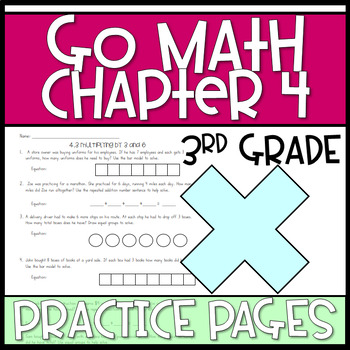# 3rd Grade Go Math Resources Chapter 4Subject
Resource Type
Common Core Standards
Product Rating
File Type

PDF (Acrobat) Document File

Be sure that you have an application to open this file type before downloading and/or purchasing.

178 KB|23 pages
Share
Product Description

This resource includes extra practice on the skills covered in this chapter of Go Math. Many worksheets can be used as an extension of the lesson, while others provide extra practice on the same type of question presented on the Go Math pages.

-4.2 practice multiplying by 5 and 10 with word problems and models

-4.3 practice multiplying by 3 and 6 with word problems and models

-Solving equations using the Distributive Property

-4.5 practice multiplying by 7 with word problems and models

-4.6 Associative Property

-4.7 Patterns on a multiplication table activity

-4.8 practice multiplying by 8 with word problems and models

-4.9 practice multiplying by 9 with word problems

-practice identifying the properties of multiplication and addition (6 pages)

-Mixed multiplication practice with word problems and models

-True/False equations with multiplication facts

Total Pages
23 pages